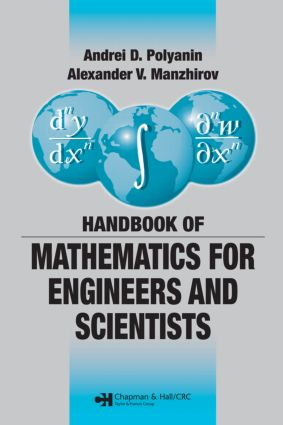# Handbook of Mathematics for Engineers and Scientists

## 1st Edition

Chapman and Hall/CRC

1,544 pages | 226 B/W Illus.

##### Purchasing Options:\$ = USD
Hardback: 9781584885023
pub: 2006-11-27
\$145.00
x
eBook (VitalSource) : 9780429143311
pub: 2006-11-27
from \$28.98

FREE Standard Shipping!

### Description

The Handbook of Mathematics for Engineers and Scientists covers the main fields of mathematics and focuses on the methods used for obtaining solutions of various classes of mathematical equations that underlie the mathematical modeling of numerous phenomena and processes in science and technology. To accommodate different mathematical backgrounds, the preeminent authors outline the material in a simplified, schematic manner, avoiding special terminology wherever possible.

Organized in ascending order of complexity, the material is divided into two parts. The first part is a coherent survey of the most important definitions, formulas, equations, methods, and theorems. It covers arithmetic, elementary and analytic geometry, algebra, differential and integral calculus, special functions, calculus of variations, and probability theory. Numerous specific examples clarify the methods for solving problems and equations. The second part provides many in-depth mathematical tables, including those of exact solutions of various types of equations.

This concise, comprehensive compendium of mathematical definitions, formulas, and theorems provides the foundation for exploring scientific and technological phenomena.

### Reviews

“The book is split into two parts: methods (about 1100 pages), and tables (about 400 pages). Both parts are well structured and well written. … the coverage of the topics included is excellent … this is a fine reference text, offered at a very reasonable price …”

SIAM Review, Vol. 49, No. 3, September 2007

Authors

Foreword

Main Notation

DEFINITIONS, FORMULAS, METHODS, AND THEOREMS

Arithmetic and Elementary Algebra

Elementary Functions

Elementary Geometry

Analytic Geometry

Algebra

Limits and Derivatives

Integrals

Series

Differential Geometry

Functions of Complex Variable

Integral Transforms

Ordinary Differential Equations

First-Order Partial Differential Equations

Linear Partial Differential Equations

Nonlinear Partial Differential Equations

Integral Equations

Difference Equations and Other Functional Equations

Special Functions and Their Properties

Calculus of Variations and Optimization

Probability Theory

Mathematical Statistics

MATHEMATICAL TABLES

Finite Sums and Infinite Series

Integrals

Integral Transforms

Ordinary Differential Equations

Systems of Ordinary Differential Equations

First-Order Partial Differential Equations

Linear Equations and Problems of Mathematical Physics

Nonlinear Mathematical Physics Equations

Systems of Partial Differential Equations

Integral Equations

Functional Equations

Supplement: Some Useful Electronic Mathematical Resources

Index

### Subject Categories

##### BISAC Subject Codes/Headings:
MAT003000
MATHEMATICS / Applied
TEC009000
TECHNOLOGY & ENGINEERING / Engineering (General)